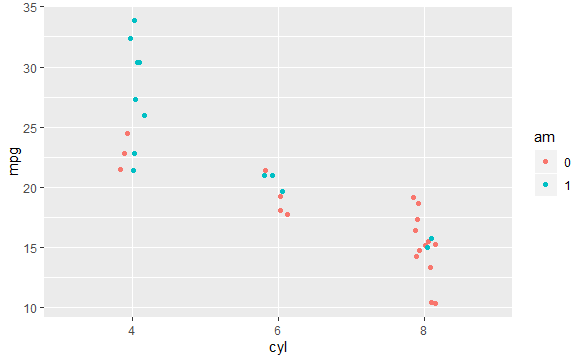3 views

I have a ggplot command

ggplot( rates.by.groups, aes(x=name, y=rate, colour=majr, group=majr))

inside a function. But I would like to be able to use a parameter of the function to pick out the column to use as color and group. I.e. I would like something like this

f <- function( column ) {

...

ggplot( rates.by.groups, aes(x=name, y=rate, colour= ??? , group=??? ) )

}

So that the column used in the ggplot is determined by the parameter. E.g. for f("majr") we get the effect of

ggplot( rates.by.groups, aes(x=name, y=rate, colour=majr, group=majr) )

but for f("gender") we get the effect of

ggplot( rates.by.groups, aes(x=name, y=rate, colour=gender, group=gender) )

Some things I tried:

ggplot( rates.by.groups, aes(x=name, y=rate, colour= columnName , group=columnName ) )

did not work. Nor did

e <- environment()

ggplot( rates.by.groups, aes(x=name, y=rate, colour= columnName , group=columnName ), environment=e )

by

To use a variable to specify column name in ggplot, you can use the following methods:

Using get(column):

f <- function( column ) {

ggplot( mtcars, aes(x=cyl, y=mpg, colour= get(column),

group=get(column) ) )+

geom_jitter(width = 0.1)

}

f("am")

Using aes_string function:

f <- function( column ) {

ggplot( mtcars, aes_string(x="cyl", y="mpg", colour= column,

group=column ))+

geom_jitter(width = 0.1)

}

f("am")

Output: